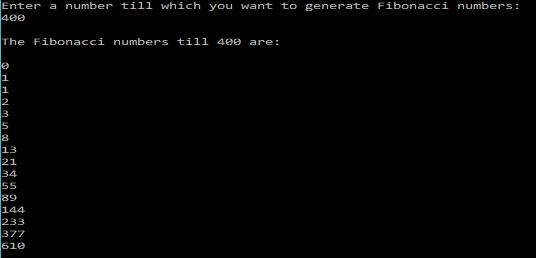# C Program to print the terms of Fibonacci Series upto a certain numberFibonacci series each member is the sum of the last two terms except the first and second term.
The following C program prints the members of the Fibonacci series upto a certain number, given by the user.

### PROGRAM:

```/***************************
*******FIBONACCI***********
2017 (c) Manas Sharma - https://bragitoff.com
**************************/
#include<stdio.h>
void fibonacci(int n){
int x;
int y;
x=0;
x=1;
printf("\n0\n1");
y=x+x;
while(y<=n){
printf("\n%d",y);
x=x;
x=y;
y=x+x;
}
}
main(){
int n;
printf("Enter a number till which you want to generate Fibonacci numbers:\n");
scanf("%d",&n);
printf("\nThe Fibonacci numbers till %d are: \n",n);
fibonacci(n);
}```

### OUTPUT:[wpedon id="7041" align="center"]